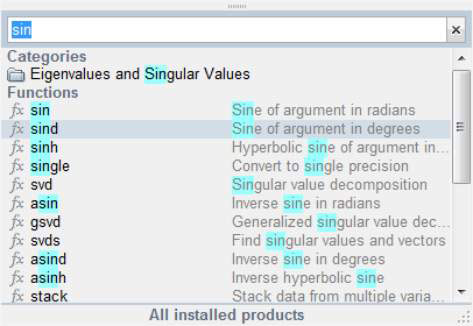##### MATLAB For Dummies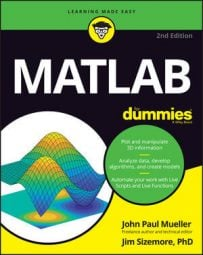It’s time to take your first steps beyond using MATLAB as a simple calculator. You can use the following MATLAB functions to perform complex tasks. Take a look to get going.

## Learning the truth

Determining whether something is true is an important part of performing most tasks. You determine the truth value of the information you receive almost automatically thousands of times a day. Computers can perform comparisons and report whether something is true (it does compare) or false (it doesn’t compare). A man named George Boole created a method for quantifying the truth value of information using Boolean logic.

The basic idea is to ask the computer to perform a comparison of two variables. Depending on the values in those variables, the computer will say that it’s either true that they compare or false that they compare. Check out how Boolean logic works within MATLAB (where an output value of 1 means the statement is true and an output value of 0 means the statement is false).

Meaning Operator Example
Less than A < B A=2;
B=3;
A==B
ans = 1
Less than or equal to A <= B A=2;
B=3;
A==B
ans = 1
Equal A == B A=2;
B=3;
A==B
ans = 0
Greater than or equal to A >= B A=2;
B=3;
A==B
ans = 0
Greater than A > B A=2;
B=3;
A==B
ans = 0
Not equal A ~= B A=2;
B=3;
A==B
ans = 1

It’s essential to remember that one equal sign (=) is an assignment operator. It assigns the value you provide to the variable. Two equal signs (==) is an equality operator. This operator determines whether two variables contain the same value.

## Using the built-in functions

MATLAB has a lot of functions beyond the basics, such as sin(), cos(), tan(), asin(), acos(), atan(), log(), and exp().

For an exhaustive list of functions, go to Appendix A. Yes, there really are that many. The appendix has brief descriptions of each function. Also, you can get additional information by typing help(‘function_name’) and pressing Enter. Try it now. Type help(‘sin') and press Enter.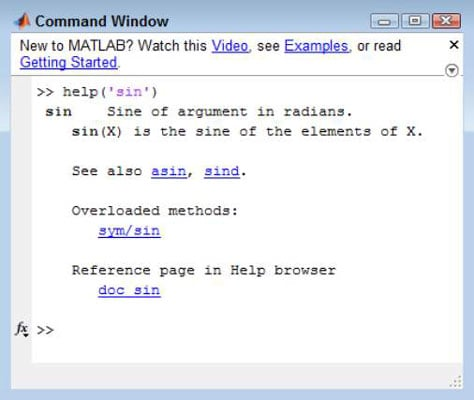## Accessing the function browser

With all the functions that MATLAB provides, you might think it’s impossible to discover what they are without a lot of memorization. Fortunately, help is closer than you might think. Look carefully at the Command window and you see an fx symbol in the border next to the prompt. Click the down arrow under the symbol and you see a dialog box.

The official name of this dialog box is the Function Browser, and you use it to browse through categories of functions to track down the function you want.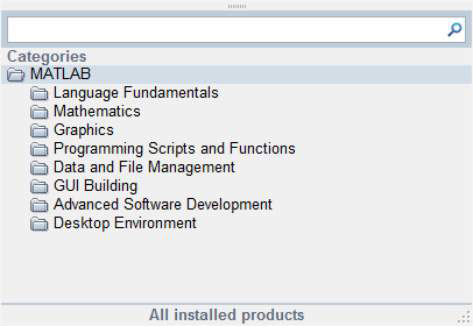You can also access the Function Browser using these techniques:

• Right-click the Command window and choose Function Browser from the context menu.

• Press Shift+F1.

Now that you have a better idea of what the Function Browser is, it’s time to look at it in more detail.

### Looking through the Function categories

The Function Browser is designed for you to easily drill down into a topic until you find precisely what you need. For example, when you click the Mathematics folder, you see a number of subcategories, such as Elementary Math, Linear Algebra, and Interpolation.

When you click Elementary Math, you see yet more subcategories, such as Arithmetic, Trigonometry, and Polynomials. When you finally get to a list of functions, you see the fx symbol next to the entries.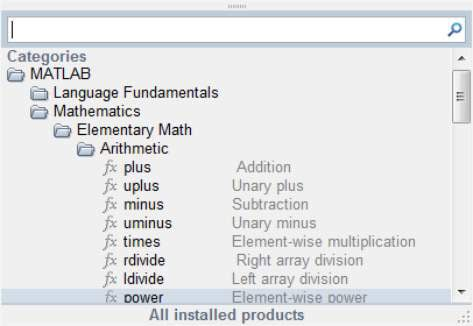### Searching for a particular function

Sometimes you already have a good idea of what you want to find. In such a case, you can type all or part of a function name in the search bar at the top of the Function Browser window. For example, type sin to see all the functions that relate to working with sine.